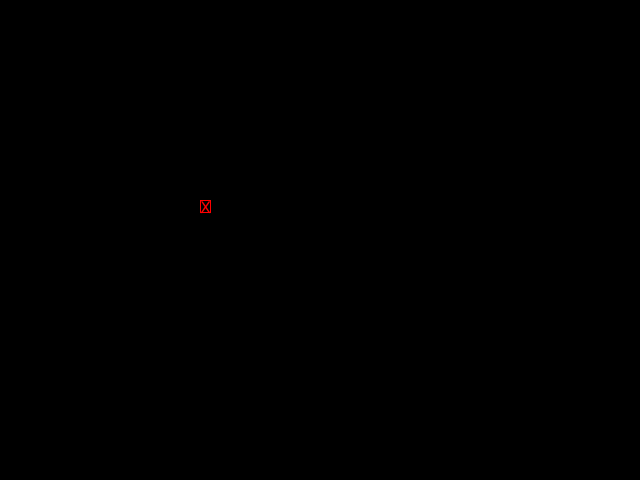# [−][src]Module plotters::element

Defines the drawing elements, the high-level drawing unit in Plotters drawing system

## Introduction

An element is the drawing unit for Plotter's high-level drawing API. Different from low-level drawing API, an element is a logic unit of component in the image. There are few built-in elements, including `Circle`, `Pixel`, `Rectangle`, `Path`, `Text`, etc.

All element can be drawn onto the drawing area using API `DrawingArea::draw(...)`. Plotters use "iterator of elements" as the abstraction of any type of plot.

You can also define your own element, `CandleStick` is a good sample of implementing complex element. There are two trait required for an element:

• `PointCollection` - the struct should be able to return an iterator of key-points under guest coordinate
• `Drawable` - the struct is a pending drawing operation on a drawing backend with pixel-based coordinate

An example of element that draws a red "X" in a red rectangle onto the backend:

```use std::iter::{Once, once};
use plotters::element::{PointCollection, Drawable};
use plotters::drawing::backend::{BackendCoord, DrawingErrorKind};
use plotters::style::IntoTextStyle;
use plotters::prelude::*;

// Any example drawing a red X
struct RedBoxedX((i32, i32));

// For any reference to RedX, we can convert it into an iterator of points
impl <'a> PointCollection<'a, (i32, i32)> for &'a RedBoxedX {
type Borrow = &'a (i32, i32);
type IntoIter = Once<&'a (i32, i32)>;
fn point_iter(self) -> Self::IntoIter {
once(&self.0)
}
}

// How to actually draw this element
impl <DB:DrawingBackend> Drawable<DB> for RedBoxedX {
fn draw<I:Iterator<Item = BackendCoord>>(
&self,
mut pos: I,
backend: &mut DB,
_: (u32, u32),
) -> Result<(), DrawingErrorKind<DB::ErrorType>> {
let pos = pos.next().unwrap();
let color = RED.to_rgba();
backend.draw_rect(pos, (pos.0 + 10, pos.1 + 12), &color, false)?;
let text_style = &("sans-serif", 20).into_text_style(backend).color(&color);
backend.draw_text("X", &text_style, pos)
}
}

fn main() -> Result<(), Box<dyn std::error::Error>> {
let root = BitMapBackend::new(
"plotters-doc-data/element-0.png",
(640, 480)
).into_drawing_area();
root.draw(&RedBoxedX((200, 200)))?;
Ok(())
}```## Composable Elements

You also have an convenient way to build an element that isn't built into the Plotters library by combining existing elements into a logic group. To build an composable element, you need to use an logic empty element that draws nothing to the backend but denotes the relative zero point of the logical group. Any element defined with pixel based offset coordinate can be added into the group later using the `+` operator.

For example, the red boxed X element can be implemented with Composable element in the following way:

```use plotters::prelude::*;
fn main() -> Result<(), Box<dyn std::error::Error>> {
let root = BitMapBackend::new(
"plotters-doc-data/element-1.png",
(640, 480)
).into_drawing_area();
let font:FontDesc = ("sans-serif", 20).into();
root.draw(&(EmptyElement::at((200, 200))
+ Text::new("X", (0, 0), &"sans-serif".into_font().resize(20.0).color(&RED))
+ Rectangle::new([(0,0), (10, 12)], &RED)
))?;
Ok(())
}```## Dynamic Elements

By default, Plotters uses static dispatch for all the elements and series. For example, the `ChartContext::draw_series` method accepts an iterator of `T` where type `T` implements all the traits a element should implement. Although, we can use the series of composable element for complex series drawing. But sometimes, we still want to make the series heterogynous, which means the iterator should be able to holds elements in different type. For example, a point series with cross and circle. This requires the dynamically dispatched elements. In plotters, all the elements can be converted into `DynElement`, the dynamic dispatch container for all elements (include external implemented ones). Plotters automatically implements `IntoDynElement` for all elements, by doing so, any dynamic element should have `into_dyn` function which would wrap the element into a dynamic element wrapper.

For example, the following code counts the number of factors of integer and mark all prime numbers in cross.

```use plotters::prelude::*;
fn num_of_factor(n: i32) -> i32 {
let mut ret = 2;
for i in 2..n {
if i * i > n {
break;
}

if n % i == 0 {
if i * i != n {
ret += 2;
} else {
ret += 1;
}
}
}
return ret;
}
fn main() -> Result<(), Box<dyn std::error::Error>> {
let root =
BitMapBackend::new("plotters-doc-data/element-3.png", (640, 480))
.into_drawing_area();
root.fill(&WHITE)?;
let mut chart = ChartBuilder::on(&root)
.x_label_area_size(40)
.y_label_area_size(40)
.margin(5)
.build_ranged(0..50, 0..10)?;

chart
.configure_mesh()
.disable_x_mesh()
.disable_y_mesh()
.draw()?;

chart.draw_series((0..50).map(|x| {
let center = (x, num_of_factor(x));
// Although the arms of if statement has different types,
// but they can be placed into a dynamic element wrapper,
// by doing so, the type is unified.
if center.1 == 2 {
Cross::new(center, 4, Into::<ShapeStyle>::into(&RED).filled()).into_dyn()
} else {
Circle::new(center, 4, Into::<ShapeStyle>::into(&GREEN).filled()).into_dyn()
}
}))?;

Ok(())
}```## Structs

 BitMapElement The element that contains a bitmap on it Boxplot The boxplot element CandleStick The candlestick data point element Circle A circle element ComposedElement The composed element which has at least two components Cross Describe a cross DynElement The container for a dynamically dispatched element EmptyElement An empty composable element, which is the start point of an ad-hoc composable element ErrorBar ErrorBarOrientH ErrorBarOrientV MultiLineText An multi-line text element. The `Text` element allows only single line text and the `MultiLineText` supports drawing multiple lines PathElement An element of a series of connected lines Pixel An element of a single pixel Polygon An element of a filled polygon Rectangle A rectangle element Text A single line text element. This can be owned or borrowed string, dependents on `String` or `str` moved into. TriangleMarker Describe a triangle marker

## Traits

 Drawable The trait indicates we are able to draw it on a drawing area IntoDynElement The trait that makes the conversion from the statically dispatched element to the dynamically dispatched element PointCollection A type which is logically a collection of points, under any given coordinate system PointElement The element that used to describe a point

## Type Definitions

 Path Deprecated